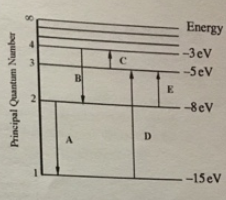# Problem: For this question consider the Figure to the right shows an energy level diagram for a certain atom (not hydrogen). Several transitions are shown and are labelled by letters. Note: The diagram is not drawn to scale. a. Which transition corresponds to the emission of the photon with the longest wavelength? b. Which transition corresponds to the emission of the photon with the shortest wavelength?

###### FREE Expert Solution

The energy of a photon:

$\overline{){\mathbf{E}}{\mathbf{=}}{\mathbf{h}}{\mathbf{f}}{\mathbf{=}}\frac{\mathbf{h}\mathbf{c}}{\mathbf{\lambda }}}$

Transitions A and B represent emissions while transitions C, D and E represent absorptions.

Energy is inversely proportional to wavelength, λ.

81% (265 ratings)###### Problem Details

For this question consider the Figure to the right shows an energy level diagram for a certain atom (not hydrogen). Several transitions are shown and are labelled by letters. Note: The diagram is not drawn to scale.a. Which transition corresponds to the emission of the photon with the longest wavelength?

b. Which transition corresponds to the emission of the photon with the shortest wavelength?